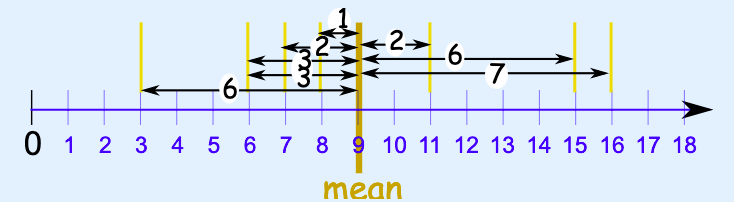# #85 Mean Absolute Deviation (MAD)image taken from https://www.mathsisfun.com/data/mean-deviation.html

### Mean Absolute Deviation (MAD) is how far, on average, all data values are from the middle.

#### To find the MAD, you can follow 3 easy steps:

1. Find the mean of the values
2. Find the distance of each value from that mean (subtract the mean from each value, ignore minus signs which is also the absolute value)
3. Then find the mean of those distances

#### For this Math Mini Spark, you’ll be finding the MAD on a spreadsheet.  Follow the steps below.

Step 1:  Make a Copy of this Spreadsheet by clicking the link: https://docs.google.com/spreadsheets/d/1ndcgCZ1EeVcKausxtkmBkONVfCeilbQNE6ukdUCWYk8/copy

Step 2:  Watch the video below and complete the steps shown in the video on your own spreadsheet.

Video: https://youtu.be/nGG2xq1COwE

NOTE:  This mini-spark can be used as 1 spreadsheet lesson for the Spreadsheet Superstar Badge.